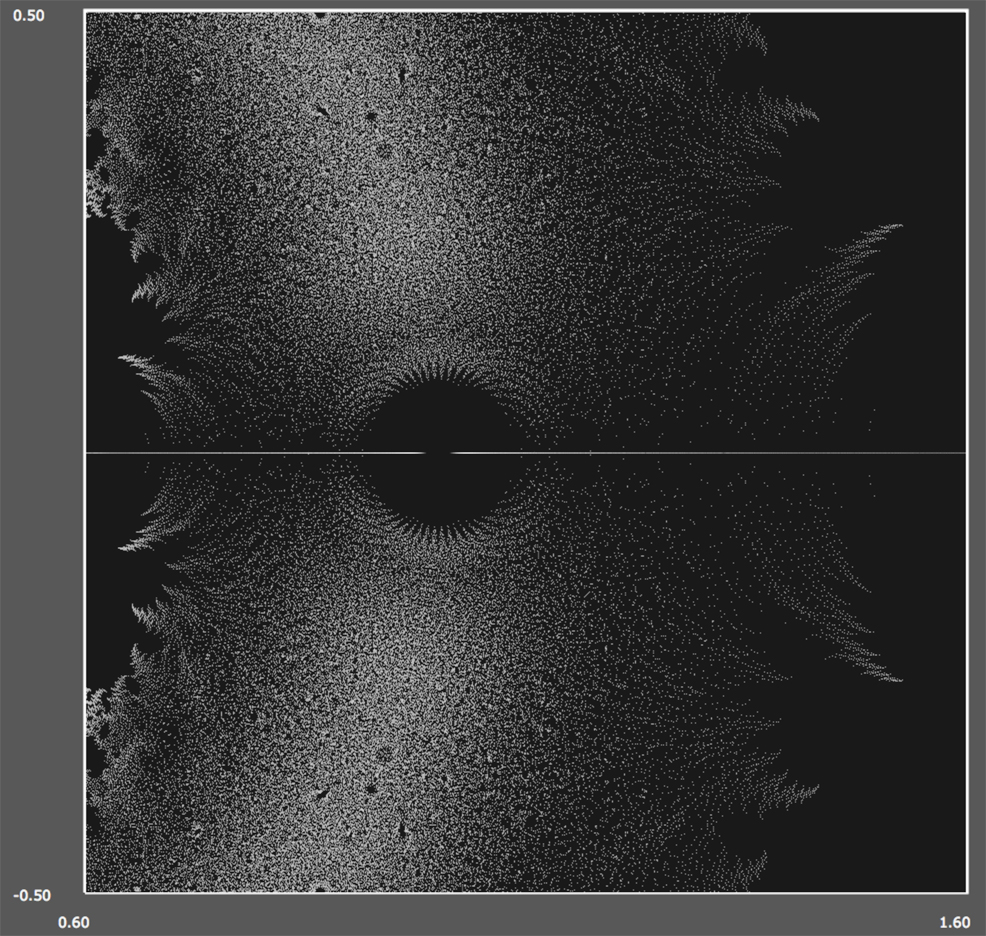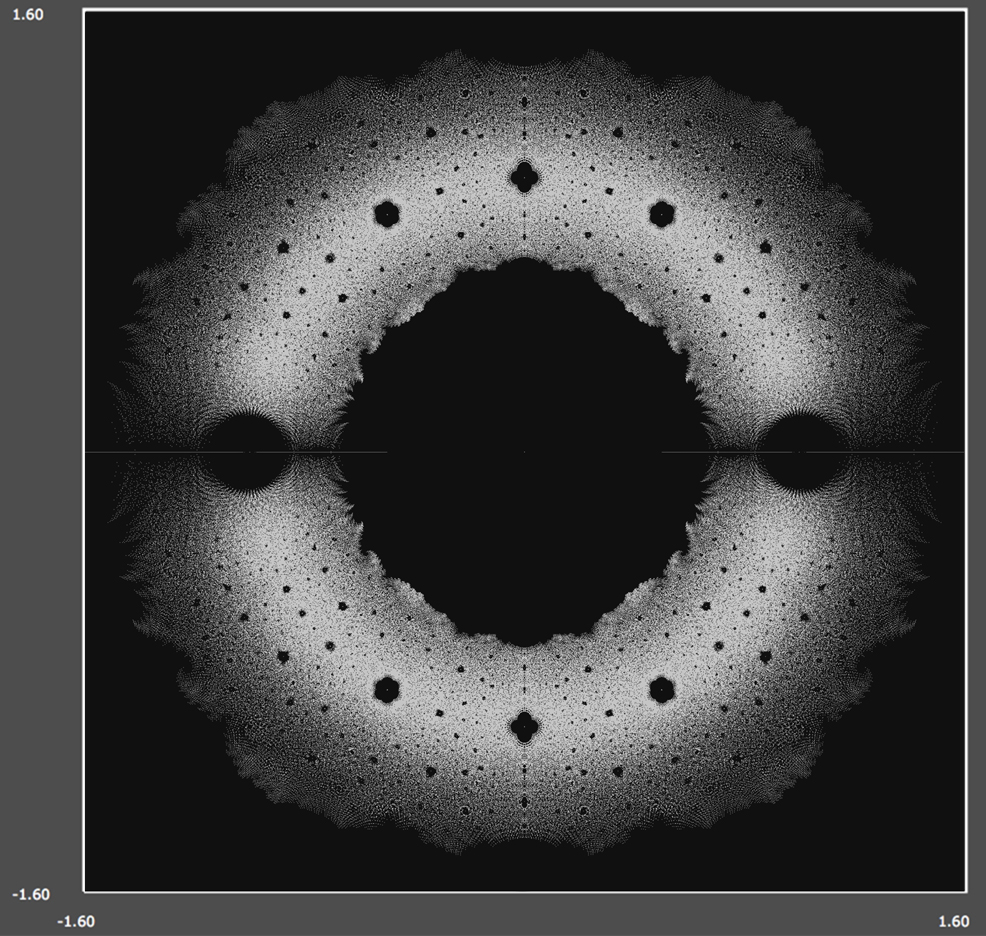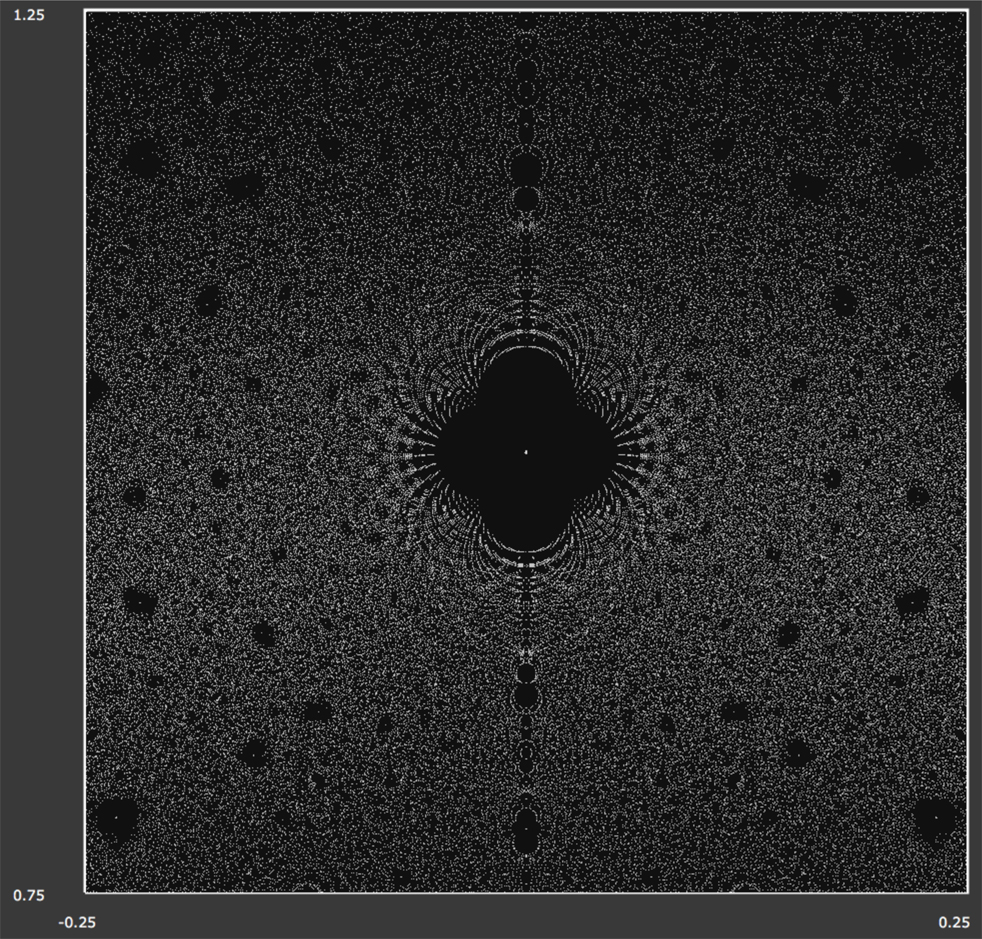## Roots of Functions: $$\quad F(z) = \displaystyle \sum_{j = 0}^n \alpha_j \; f_j(z)$$   where   $$\alpha_j \in \{ -1, +1 \}$$

Scroll Down For Some Screen Shots

$$f_j(z)$$ = ,       n = ,     $$\alpha_i$$'s =

Size =     x center =     y center =

Renormalize intensity for real and complex roots together?

This app computes and plots all roots of functions of the form

$$F(z) = \displaystyle \sum_{j = 0}^n \alpha_j \; f_j(z)$$

for which all the coefficients $$\alpha_j$$'s are either $$+1$$ or $$-1$$ and the functions $$f_j(z)$$ are arbitrary analytic functions on the complex plane. The functions $$f_j(z)$$ can be selected using the pull-down menu above. The default choice of $$f_j(z) = z^j$$ gives us $$n$$-th degree polynomials. The number of terms, $$n$$, can also be changed.

Here's my Matlab version of the code:   http://vanderbei.princeton.edu/WebGL/PlusMinusOne.m

Here's a related webpage by Dan Christensen:   http://jdc.math.uwo.ca/roots/
Here's a related webpage by John Baez:   http://math.ucr.edu/home/baez/roots/

### Here's a screenshot for the $$18$$-degree polynomial case...### Here's a screenshot for a closeup view of the $$18$$-degree polynomial case...### Here's a screenshot for a closeup view of the $$16$$-degree polynomial case...### Here's a screenshot for the $$12$$-degree polynomial case with +1,0,-1 coefficients...### Here's a screenshot for a closeup view of the $$12$$-degree polynomial case with +1,0,-1 coefficients...Updated 2017 Oct 25